# MA.912.GR.1.3Export Print
Prove relationships and theorems about triangles. Solve mathematical and real-world problems involving postulates, relationships and theorems of triangles.

### Clarifications

Clarification 1: Postulates, relationships and theorems include measures of interior angles of a triangle sum to 180°; measures of a set of exterior angles of a triangle sum to 360°; triangle inequality theorem; base angles of isosceles triangles are congruent; the segment joining midpoints of two sides of a triangle is parallel to the third side and half the length; the medians of a triangle meet at a point.

Clarification 2: Instruction includes constructing two-column proofs, pictorial proofs, paragraph and narrative proofs, flow chart proofs or informal proofs.

Clarification 3: Instruction focuses on helping a student choose a method they can use reliably.

General Information
Subject Area: Mathematics (B.E.S.T.)
Strand: Geometric Reasoning
Status: State Board Approved

## Benchmark Instructional Guide

### Terms from the K-12 Glossary

• Angle
• Isosceles Triangle
• Triangle

### Vertical Alignment

Previous Benchmarks

Next Benchmarks

### Purpose and Instructional Strategies

In grade 8, students solved problems involving right triangles, including using the Pythagorean Theorem, and angle measures within triangles. In Geometry, students prove relationships and theorems about triangles and solve problems involving triangles, including right triangles. In later courses, students will use vectors and trigonometry to study and prove further relationships between angle measures and side lengths of triangles.
• While the focus of this benchmark are the postulates, relationships and theorems listed in Clarification 1, instruction could include other definitions, postulates, relationships or theorems like a midsegment of a triangle and other angle or side length measures, the Hinge Theorem and the Scalene Triangle Inequality Theorem. Additionally, instruction includes the converse (i.e., if conclusion, then hypothesis) of some postulates and theorems.
• Instruction includes the connection to the Logic and Discrete Theory benchmarks when developing proofs. Additionally, with the construction of proofs, instruction reinforces the Properties of Operations, Equality and Inequality. (MTR.5.1)
• Instruction utilizes different ways students can organize their reasoning by constructing various proofs when proving geometric statements. It is important to explain the terms statements and reasons, their roles in a geometric proof, and how they must correspond to each other. Regardless of the style, a geometric proof is a carefully written argument that begins with known facts, proceeds from there through a series of logical deductions, and ends with the statement you are trying to prove. (MTR.2.1
• For examples of different types of proofs, please see MA.912.LT.4.8
• Instruction includes the connection to compass and straight edge constructions and how the validity of the construction is justified by a proof. (MTR.5.1)
• Students should develop an understanding for the difference between a postulate, which is assumed true without a proof, and a theorem, which is a true statement that can be proven. Additionally, students should understand why relationships and theorems can be proven and postulates cannot.
• Instruction includes the use of hatch marks, hash marks, arc marks or tick marks, a form of mathematical notation, to represent segments of equal length or angles of equal measure in diagrams and images.
• Students should understand the difference between congruent and equal. If two segments are congruent (i.e., PQMN), then they have equivalent lengths (i.e., PQ = MN) and the converse is true. If two angles are congruent (i.e., ∠ABC ≅ ∠PQR), then they have equivalent angle measure (i.e., $m$ABC = $m$PQR) and the converse is true.
• Instruction includes the use of hands-on manipulatives and geometric software for students to explore relationships, postulates and theorems.
• When proving the Pythagorean Theorem, instruction includes making the connection to similarity criterion.
• For example, given triangle ABC with $m$C = 90° and $h$, the height to the hypotenuse, students can begin the proof that $a$2 + $b$2 = $c$by first proving that ΔAHC~ΔCHB~ΔACB using the Angle-Angle (AA) criterion. Students should be able to conclude that, from the definition of similar triangles, $\frac{\text{a}}{\text{c}}$ = $\frac{\text{m}}{\text{a}}$ (in ΔAHC~ΔACB) and $\frac{\text{b}}{\text{c}}$ = $\frac{\text{n}}{\text{b}}$ (in ΔCHB~ΔACB). Students can equivalently rewrite these equations as  $a$2 = $c$$m$ and $b$2 = $c$$n$. Then students can add the resulting equations to create $a$2 + $b$2 = $c$$m$ + $c$$n$. Using algebraic reasoning the segment addition postulate, students can conclude that $a$2 + $b$2 = $c$2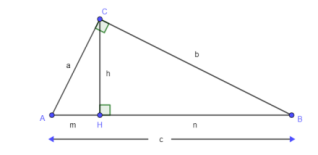• Instruction includes determining if a triangle can be formed from a given set of sides. The instruction in this benchmark includes discussing the converses of the Triangle Inequality Theorem and the Pythagorean Theorem to understand whether a triangle or a right triangle can be formed from three sides with given side lengths.
• In triangle ABC, with sides $a$, $b$ and $c$, opposite to A, B and C respectively, applying the Triangle Inequality Theorem: $a$ + $b$ > $c$, $b$ + $c$ > $a$ and $a$ + $c$ > $b$. From $a$ + $b$ > $c$, $c$ < $a$ + $b$. From $b$ + $c$ > $a$, $c$ > $a$$b$ or $a$$b$ < $c$. Combining the resulting inequalities: $a$$b$ < $c$ < $a$ + $b$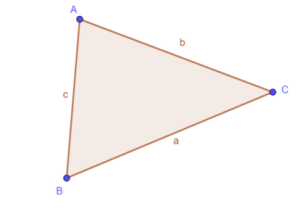• For example, in a triangle with sides $a$, $b$ and $c$, with $c$ being the longest one, if  $a$2 + $b$2 = $c$2, then the triangle is right. But if $a$2 + $b$2 > $c$2, then the triangle is acute, and if $a$2 + $b$ < $c$2, then the triangle is obtuse.
• Instruction includes the understanding of the Scalene Triangle Inequality Theorem (the angle opposite the longer side in a triangle has greater measure), the converse of the Scalene Triangle Inequality Theorem (the side opposite the greater angle in a triangle is longer), and the Hinge Theorem (if two sides of two triangles are congruent and the included angle is different, then the angle that is larger is opposite the longer side).
• For example, given triangle ABC where AB > AC, students can begin the proof of the Scalene Triangle Inequality Theorem by placing the point P such that AP = AC. Then, $m$∠1 = $m$∠2. As $m$ACB > $m$∠2, $m$ACB > $m$∠1 by the substitution property. ∠1 is exterior to triangle BPC, so $m$∠1 = $m$∠3 + $m$∠4 and $m$∠1 > $m$∠3. Since $m$∠ACB > $m$∠1 and $m$∠1 > $m$∠3, $m$ACB > $m$∠3 or $m$ACB > $m$ABC. From this we can conclude, if AB > AC then $m$ACB > $m$ABC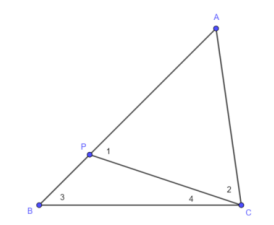• For example, given triangle ABC where $m$ACB > $m$ABC students can prove the converse of the Scalene Triangle Inequality Theorem by using contradiction to show that AB > AC. Students can realize that if AC > AB, then the Scalene Triangle Inequality Theorem would imply that $m$ABC > $m$ACB contradicting the given information. Additionally, students can realize that if  AB = AC, then $m$ABC =  m∠ABC, which is another contradiction of the given information. Therefore, students can conclude that AB > AC• For example, given triangles ABD and ACB where AB = AC and $m$BAD > $m$CAD, students can begin the proof of the Hinge Theorem by identifying that triangle ABC is an isosceles triangle with AB = AC and $m$ABC = $m$ACB. Students should realize that $m$DCB > $m$ACB, which is the same as $m$DCB > $m$ABC. Since $m$ABC > $m$DBC, students can conclude that $m$DCB > $m$DBC. Applying the Scalene Triangle Inequality Theorem to triangle BCD, students can conclude that DB > DC.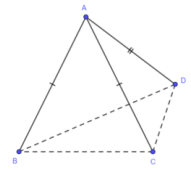• Instruction includes making the connection to parallel lines and their angle relationships to proving the measures of the interior angles of a triangle sum to 180°.
• For example, given triangle ABC, students can construct a line through the point B that is parallel AC. Students can then explore the relationships between the angle measures in the image below. Students should realize that $m$∠4 + $m$∠2 + $m$∠5 = 180°, and $m$∠1 = $m$∠4 and $m$∠3 = $m$∠5 (Alternate Interior Angles Theorem). Applying the Substitution Property of Equality, students should be able to conclude that $m$∠1 + $m$∠2 + $m$∠3 = 180°.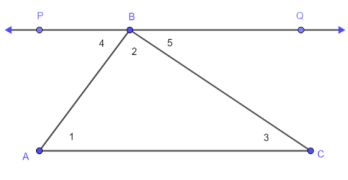• Instruction includes discussing the relationship between the Triangle Sum Theorem and an exterior angle of a triangle and its two remote interior angles.
• For example, given triangle ABC, students should realize that $m$∠4 = $m$∠1 + $m$∠2.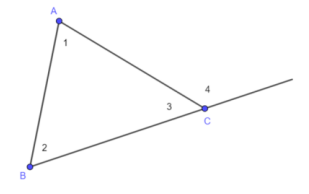• Instruction for the proof that the measures of a set of exterior angles of a triangle sum to 360° includes the connection to algebraic reasoning skills, the Triangle Sum Theorem and properties of equality.
• For example, given the triangle below, students should be able to realize that $m$∠1 + $m$∠4 + $m$∠2 + $m$∠5 + $m$∠3 + $m$∠6 = 540° since there are three pairs of linear pair angles. Applying properties of equalities and the Triangle Sum Theorem, students should be able to conclude that $m$∠4 + $m$∠5 + $m$∠6 = 360°.
• The proof of the Triangle Inequality Theorem can be approached in a variety of ways. Instruction includes the connection to the Pythagorean Theorem.
• For example, students can first use the Pythagorean Theorem to prove that the hypotenuse of a right triangle is longer than each of the two legs of the right triangle. Given triangle ABC with an altitude CH, students can realize that there are two right triangles ACH and BCH; with AC as the hypotenuse of ΔACH and CB is the hypotenuse of ΔBCH. Students can use their knowledge of right triangles to determine that AC > AH and AC > HC, and CB > HB and CB > CH. By adding two of the inequalities, AC > AH and CB > HB, students can determine that AC + CB > AH + HB which is equivalent to AC + CB > AB by
• When proving the Isosceles Triangle Theorem, instruction includes constructing an auxiliary line segment (e.g., median, altitude or angle bisector) from its base to the opposite vertex. (MTR.2.1
• For example, given triangle ABC with ACBC, student can construct the median from point C to side AB, with point of intersection M. Students can use the definition of the median of a triangle to state that AMMC. Students should be be able to realize that ΔAMC ΔBMC by Side-Side-Side (SSS). So, ∠A ≅ ∠B since corresponding parts of congruent triangles are congruent (CPCTC).
• When proving the Triangle Midsegment Theorem, instruction includes the connection to coordinate geometry or to triangle congruence and properties of parallelograms.
• For example, given triangle ABC on the coordinate plane with A at the origin, B at the point ($b$, 0) and C at point ($x$, $y$). Students can determine the midpoint of AC at the point P, ($\frac{\text{1}}{\text{2}}$$x$, $\frac{\text{1}}{\text{2}}$$y$) and the midpoint of  CB at the point Q,($\frac{\text{x + b}}{\text{2}}$, $\frac{\text{1}}{\text{2}}$$y$) Students should realize that PQ is horizontal and is parallel to the base of the triangle, AB. To determine the length of PQ, students can subtract the $x$-coordinates of P and Q to find it has a length of $\frac{\text{1}}{\text{2}}$$b$. Since PQ = $\frac{\text{1}}{\text{2}}$$b$ and AB = $b$, then PQ = $\frac{\text{1}}{\text{2}}$AB and that AB = 2(PQ).
• Instruction includes the connection between the Triangle Midsegment Theorem and the Trapezoid Midsegment Theorem. (MTR.5.1)
• For example, students can start with a trapezoid and its midsegment then using geometric software, shrink the top base until it has zero length producing a triangle. Students should be able to realize that the average of the lengths of the two bases of the trapezoids becomes one-half the length of the base of the triangle.
• When proving the medians of a triangle meet in a point, instruction includes the connection to coordinate geometry or to the Midpoint Segment Theorem.
• For example, given triangle ABC, students can prove that all medians meet at the same point by first constructing two medians, AQ and BP intersecting at the point S. Students should realize that the segment PQ is a midpoint segment. By the Midpoint Segment Theorem, PQ is parallel to AB, therefore students can use the Angle-Angle-Angle (AAA) criterion to state that triangles ASB and QSP are similar. Also, by the Midpoint Segment Theorem, 2PQ = AB. So the scale factor between the two triangles is 2 and students can conclude that AS = 2QS and that point S is the 2:1 partition point of AQ.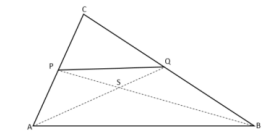Students can next look at the median from the point C that intersects AB at point R. Using the same procedure as above, students can prove that median CR also intersects the median AQ at the 2:1 partition point S. Therefore, all three medians go through the point S.
• For example, students can explore and prove facts about medians of a triangle using the midpoint formula and equations of lines. Students can write the equations of the lines containing two medians and solve the system of equations to determine the point of intersection of the two medians (the centroid). To prove the three medians meet at that point, students can show the centroid is a solution to the equation of the line containing the third median.
• For example, students can use the notion of the weighted average of two points and its connection to the partitioning of line segments to explore and prove facts about medians. The Centroid Theorem states that the centroid partitions the median from the vertex to the midpoint of the opposite side in the ratio 2: 1. In other words, the centroid is $\frac{\text{2}}{\text{3}}$ of the way from the vertex to the midpoint of the opposite side. This fact about the centroid can also be applied to show how the medians meet at a point.

### Common Misconceptions or Errors

• Students may extend two sides of a triangle when using exterior angles. An exterior angle of a triangle is formed by the extension of one side of the triangle, not two.

• Directions: Print and cut apart the given information, statements and reasons for the proof and provide to students. Students can work individually or in groups. Additionally, students can develop the proof with or without all of the intermediate steps.

Instructional Task 2 (MTR.2.1, MTR.4.1, MTR.5.1
• Provide students with various sizes and types of triangles cut from a paper; large enough for students to tear off the vertices of the triangles. Additionally, provide students tape, glue stick and blank piece of paper.
• Part A. Using one of the triangles provided, tear off the vertices.
• Part B. Place the three vertices in such a way that they are adjacent and create a straight line. If necessary, use tape or glue to keep the vertices in place on the straight line.
• Part C. What do you notice about the type of angle the three vertices create? If each of the angle measured are added together, how many degrees does it sum to?
• Part D. How does this relate to the Triangle Sum Theorem?

Instructional Task 3 (MTR.2.1, MTR.4.1, MTR.5.1
• Given triangle ABC and its medians shown in the figure, prove that they meet in a point, P.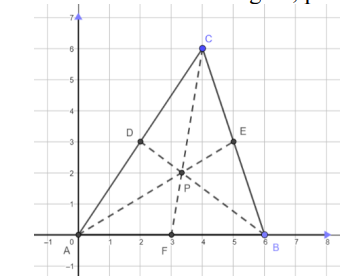• Part A. Find the midpoints of the three sides of the triangle ABC (D, E and F).
• Part B. Write the equations of the lines containing two of the medians of the triangle.
• Part C. Find the solution of the system of equations created from Part B. Compare your solution with a partner.
• Part D. Write the equation of the line containing the third median of the triangle.
• Part E. Check that the solution found from Part C satisfies the equation from Part D. If so, what can you conclude about the three medians of the triangle?

### Instructional Items

Instructional Item 1
• GH is a midsegment of triangle DEF and DE is a midsegment of triangle ABC. If GH = 1.5 cm, what is the length of segment BC?.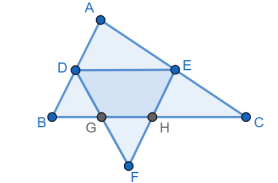*The strategies, tasks and items included in the B1G-M are examples and should not be considered comprehensive.

## Related Courses

This benchmark is part of these courses.
1200400: Foundational Skills in Mathematics 9-12 (Specifically in versions: 2014 - 2015, 2015 - 2022, 2022 and beyond (current))
1206310: Geometry (Specifically in versions: 2014 - 2015, 2015 - 2022, 2022 and beyond (current))
1206320: Geometry Honors (Specifically in versions: 2014 - 2015, 2015 - 2022, 2022 and beyond (current))
1206315: Geometry for Credit Recovery (Specifically in versions: 2014 - 2015, 2015 - 2022, 2022 and beyond (current))
7912065: Access Geometry (Specifically in versions: 2015 - 2022, 2022 and beyond (current))

## Related Access Points

Alternate version of this benchmark for students with significant cognitive disabilities.
MA.912.GR.1.AP.3: Use the relationships and theorems about triangles. Solve mathematical and/or real-world problems involving postulates, relationships and theorems of triangles.

## Related Resources

Vetted resources educators can use to teach the concepts and skills in this benchmark.

## Formative Assessments

The Measure of an Angle of a Triangle:

Students are given the measure of one interior angle of an isosceles triangle and are asked to find the measure of another interior angle.

Type: Formative Assessment

Proving the Triangle Inequality Theorem:

Students are asked to prove the Triangle Inequality Theorem.

Type: Formative Assessment

An Isosceles Trapezoid Problem:

Students are asked to explain why the sum of the lengths of the diagonals of an isosceles trapezoid is less than its perimeter.

Type: Formative Assessment

Triangles and Midpoints:

Students are asked to explain why a quadrilateral formed by drawing the midsegments of a triangle is a parallelogram and to find the perimeter of the triangle formed by the midsegments.

Type: Formative Assessment

Interior Angles of a Polygon :

Students are asked to explain why the sum of the measures of the interior angles of a convex n-gon is given by the formula (n – 2)180°.

Type: Formative Assessment

The Third Side of a Triangle:

Students are given the lengths of two sides of a triangle and asked to describe all possible lengths of the remaining side.

Type: Formative Assessment

Name That Triangle:

Students are asked to describe a triangle whose vertices are the endpoints of a segment and a point on the perpendicular bisector of a segment.

Type: Formative Assessment

Locating the Missing Midpoint:

Students are given a triangle in which the midpoints of two sides are shown and are asked to describe a method for locating the midpoint of the remaining side using only a straight edge and pencil.

Type: Formative Assessment

Pythagorean Theorem Proof:

Students are asked to prove the Pythagorean Theorem using similar triangles.

Type: Formative Assessment

Geometric Mean Proof:

Students are asked to prove that the length of the altitude to the hypotenuse of a right triangle is the geometric mean of the lengths of the two segments of the hypotenuse.

Type: Formative Assessment

Converse of the Triangle Proportionality Theorem:

Students are asked to prove that if a line intersecting two sides of a triangle divides those two sides proportionally, then that line is parallel to the third side.

Type: Formative Assessment

Triangle Proportionality Theorem:

Students are asked to prove that a line parallel to one side of a triangle divides the other two sides of the triangle proportionally.

Type: Formative Assessment

Justifying the Triangle Sum Theorem:

Students are asked to provide an informal justification of the Triangle Sum Theorem.

Type: Formative Assessment

Median Concurrence Proof:

Students are asked to prove that the medians of a triangle are concurrent.

Type: Formative Assessment

Triangle Sum Proof:

Students are asked prove that the measures of the interior angles of a triangle sum to 180°.

Type: Formative Assessment

Isosceles Triangle Proof:

Students are asked to prove that the base angles of an isosceles triangle are congruent.

Type: Formative Assessment

## Lesson Plans

Triangles: Finding Interior Angle Measures:

The lesson begins with a hands-on activity and then an experiment with a GeoGebra-based computer model to discover the Triangle Angle Sum Theorem. The students write and solve equations to find missing angle measures in a variety of examples.

Type: Lesson Plan

Right turn, Clyde!:

Students will develop their knowledge of perpendicular bisectors & point of concurrency of a triangle, as well as construct perpendicular bisectors through real world problem solving with a map.

Type: Lesson Plan

Halfway to the Middle!:

Students will develop their knowledge of mid-segments of a triangle, construct and provide lengths of mid-segments.

Type: Lesson Plan

Location, Location, Location, Location?:

Students will use their knowledge of graphing concurrent segments in triangles to locate and identify which points of concurrency are associated by location with cities and counties within the Texas Triangle Mega-region.

Type: Lesson Plan

## Original Student Tutorial

Use properties, postulates, and theorems to prove a theorem about a triangle. In this interactive tutorial, you'll also learn how to prove that a line parallel to one side of a triangle divides the other two proportionally.

Type: Original Student Tutorial

## MFAS Formative Assessments

An Isosceles Trapezoid Problem:

Students are asked to explain why the sum of the lengths of the diagonals of an isosceles trapezoid is less than its perimeter.

Converse of the Triangle Proportionality Theorem:

Students are asked to prove that if a line intersecting two sides of a triangle divides those two sides proportionally, then that line is parallel to the third side.

Geometric Mean Proof:

Students are asked to prove that the length of the altitude to the hypotenuse of a right triangle is the geometric mean of the lengths of the two segments of the hypotenuse.

Interior Angles of a Polygon :

Students are asked to explain why the sum of the measures of the interior angles of a convex n-gon is given by the formula (n – 2)180°.

Isosceles Triangle Proof:

Students are asked to prove that the base angles of an isosceles triangle are congruent.

Justifying the Triangle Sum Theorem:

Students are asked to provide an informal justification of the Triangle Sum Theorem.

Locating the Missing Midpoint:

Students are given a triangle in which the midpoints of two sides are shown and are asked to describe a method for locating the midpoint of the remaining side using only a straight edge and pencil.

Median Concurrence Proof:

Students are asked to prove that the medians of a triangle are concurrent.

Name That Triangle:

Students are asked to describe a triangle whose vertices are the endpoints of a segment and a point on the perpendicular bisector of a segment.

Proving the Triangle Inequality Theorem:

Students are asked to prove the Triangle Inequality Theorem.

Pythagorean Theorem Proof:

Students are asked to prove the Pythagorean Theorem using similar triangles.

The Measure of an Angle of a Triangle:

Students are given the measure of one interior angle of an isosceles triangle and are asked to find the measure of another interior angle.

The Third Side of a Triangle:

Students are given the lengths of two sides of a triangle and asked to describe all possible lengths of the remaining side.

Triangle Proportionality Theorem:

Students are asked to prove that a line parallel to one side of a triangle divides the other two sides of the triangle proportionally.

Triangle Sum Proof:

Students are asked prove that the measures of the interior angles of a triangle sum to 180°.

Triangles and Midpoints:

Students are asked to explain why a quadrilateral formed by drawing the midsegments of a triangle is a parallelogram and to find the perimeter of the triangle formed by the midsegments.

## Original Student Tutorials Mathematics - Grades 9-12

Use properties, postulates, and theorems to prove a theorem about a triangle. In this interactive tutorial, you'll also learn how to prove that a line parallel to one side of a triangle divides the other two proportionally.

## Student Resources

Vetted resources students can use to learn the concepts and skills in this benchmark.